Disclaimer: This dissertation has been written by a student and is not an example of our professional work, which you can see examples of here.

Any opinions, findings, conclusions, or recommendations expressed in this dissertation are those of the authors and do not necessarily reflect the views of UKDiss.com.

# Generative Adversarial Networks and its Application in Estimating Communication Channels

Info: 2548 words (10 pages) Dissertation
Published: 1st Dec 2021

Tagged: Physiology

## Abstract

Generative Adversarial Networks (GAN) belong to a class of Neural Networks that try to directly model the implicit density in Generative Models. In this paper we try to briefly explain What a GAN is, why it’s important and some potential applications in estimating complex communication channels. General Real-World Communication Channels are extremely tough to estimate because of the number of factors involved and are difficult to model by using only simple theoretical models. Thus, mechanisms which could effectively approximate these complex environments will help us better understand the medium, propose more complex models and test prototype devices. The Generative nature of GAN’s can be leveraged to serve this purpose and effectively estimate complex communication environments.

## I. Introduction

All of Classification Models can be divided into two types, Discriminative Models and Generative Models. Discriminative models do not have an innate understanding of the individual classes/concepts that you are trying to distinguish between and rather focus on understanding the features & attributes that differentiate them from others. On the Contrary, Generative Models try to understand the distribution of a class and predict a sample based on the similarity to the class.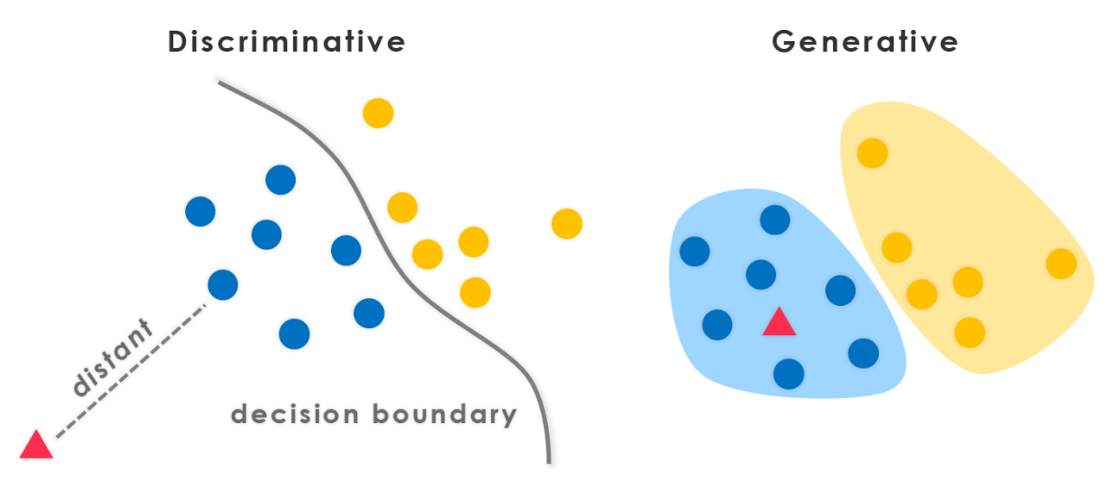Figure 1: Discriminative vs Generative Models. Source: Adapted from 

Figure 1. shows an illustration of how a potential sample is classified. In a discriminative model a decision boundary parametrized by the differentiating features dictates the class of a new sample. Whereas in a Generative model there is an understanding of the class distribution and a new sample is assigned only if it closely aligns with this distribution.

Generative Adversarial Networks fall in the Generative Models category because they try to model the underlining class instead of maximizing the dissimilarities between two different classes. Generative Models can be further divided into Explicit Density Estimators and Implicit Density estimators. Models which follow the Explicit density estimation assume some prior distribution of the data and try to parametrize it as a random variable. Networks like Variational Auto-Encoders are major examples who follow this route of explicit density estimation. Alternatively, Implicit density estimation do not assume any prior knowledge about the class and try to directly estimate the data. GAN’s are a big example of implicit density estimators, who try to generate data by pitting two Neural Networks against each other.

## II. What are Generative Adversarial Networks? Why are they important?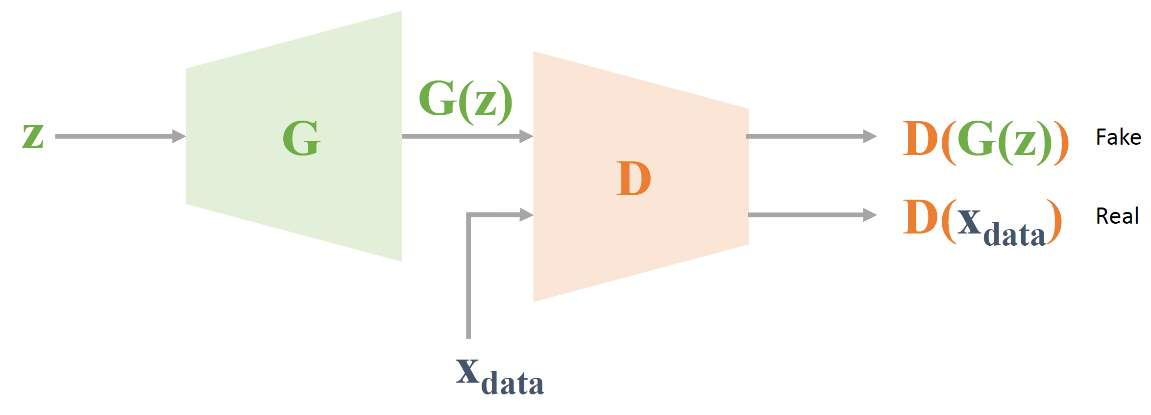Figure 2: GAN schematic. Source: Adapted from 

Generative adversarial networks have two competing neural networks which are trying to accomplish two different things as summarized in Figure 2.

The Neural Network labeled as G is called the Generator which tries to generate samples G(z) based on an input z which usually is a random noise. The network labeled as D is called the Discriminator which consumes both the generated data G(z) as well as real data Xdata and tries to classify if the input is real or fake. So, the objectives of both the networks are ‘Adversarial’ in nature, where the Generator tries to generate the most authentic fake data, and the Discriminator tries to classify real and fake. This dichotomy when trained over multiple iterations helps the whole network get better and enables the Generator to create realistic fake data without any prior understanding about the class distributions.

This makes GAN a very powerful tool in creating fake channel environments that can simulate real-world complex channels. In T. J. O’Shea et al.  a GAN was used to estimate a complex stochastic channel with no memory effects. These promising results inspired the authors (A. Smith et al.)  to design an improved GAN architecture that can model a complex communication channel which had non-linearities, non-gaussian elements and memory effects.

## III. Channel Environments, Losses and GAN Architecture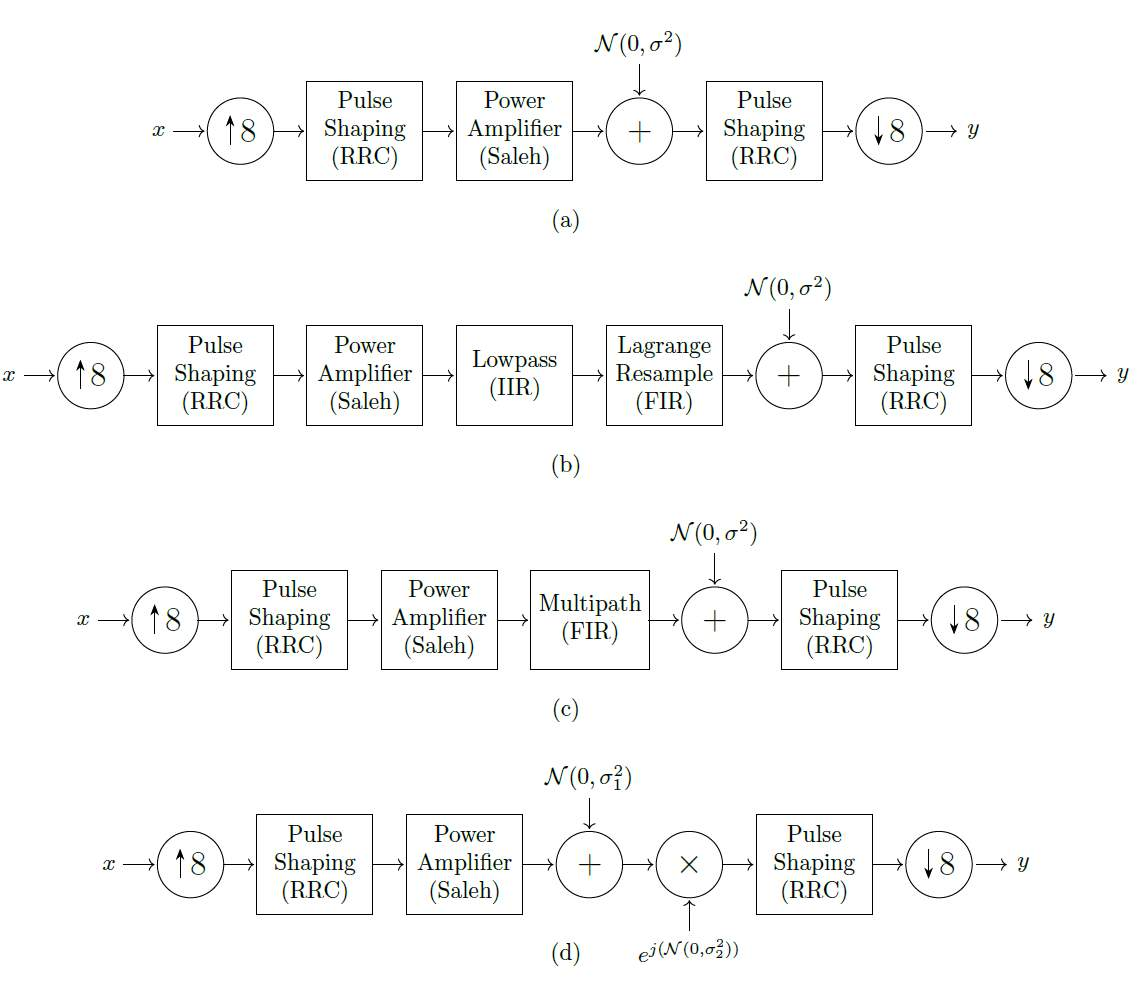Figure 3: Channel Simulations. Source: Adapted from 

To properly test the GAN architecture, they tried to create diverse channel simulations as listed in Figure 3. All the channels contain inter symbol interference and other real-world factors like non-linearities, multi-path, non-gaussian elements and other dispersive channel related issues. Figure 3. shows channel (a) which works as the default model where a symbol stream input ‘x’ undergoes Pulse Shaping followed by a memoryless amplification, addition of a Gaussian noise and finally, we have a pule shaping filter to extract the received symbols. In channel (b) they build on the default channel (a) and try to simulate a transponder that maximizes the usable spectrum which might in turn induce a group delay effect. In channel (c) they simulate a Finite Impulse Response like multi-path model and channel (d) simulates non-gaussian elements by adding an uncorrelated phase noise to the channel.

So, they tried to design an improved GAN which can estimate a real-world channel and learn its conditional probability density function. Figure 4. describes the GAN architecture which has all the components that we described before, as well as a new Encoder module which is popular among Variational Auto Encoders architectures, an explicit density based Generative model.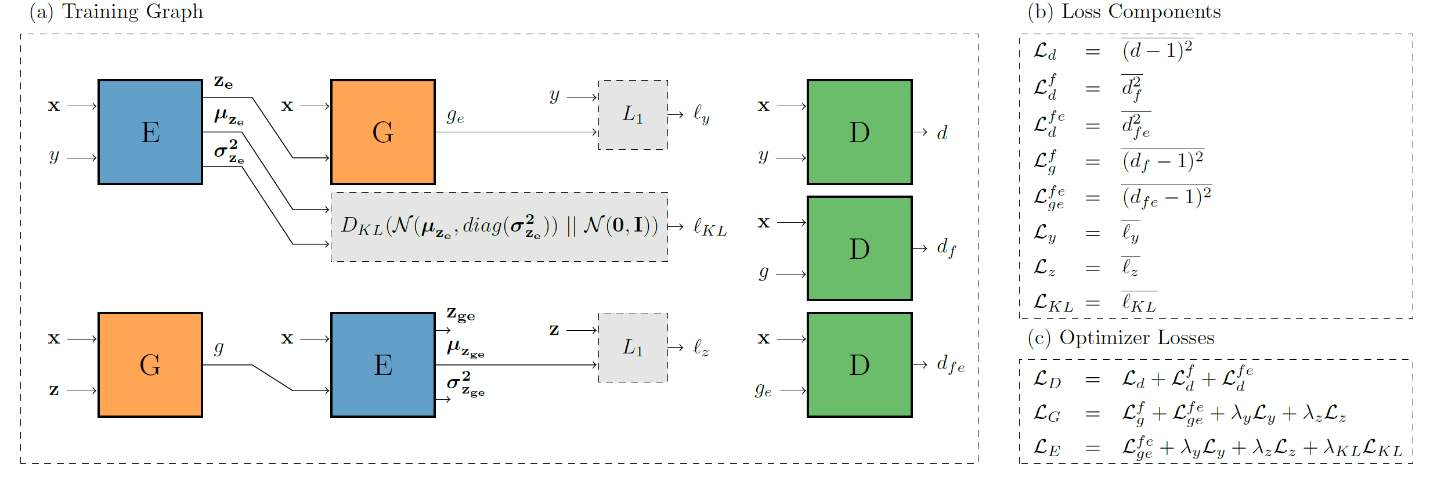Figure 4: GAN Architecture & Losses. Source: Adapted from 

Figure 4. shows the Encoder module labeled E which takes in the input x with symbols sampled from amplitude & phase-shift keying modulation scheme. The encoder tries to guess the distribution of these symbols and outputs a mean µz and variance σ2z which are then plugged into a Generator Neural Network. And the generator output plugs into the encoder completing a VAE cycle, both the half’s of the cycle are trained using two loss metrics ℓy which is the output reconstruction loss and ℓz  a latent reconstruction loss metric. The output of the Generator is then plugged into the Discriminator Neural Network labeled D, which gives out a binary output of 1 if the channel is real or 0 if it’s fake. The encoder also employs a “Kullback–Leibler divergence” metric which is a staple of the VAE machines, meant to measure the similarity between two distributions of the input space and the generated space. Typically for two distributions p and q, the KL divergence is parametrized by an integral of the form,

Consequently, the most important loss function of the Encoder ℒ KL tries to force the encoder output to a distribution closer to the latent space encountered during interference. All the respective losses for the encoder ℒ E, generator ℒ G, and discriminator ℒ D are shown in the Figure 4.

The architecture of the Generator, Discriminator and Encoder is shown in Figure 5. There is an overall energy calculation per symbol in each component, which is added to the set of inputs. The inputs are then passed into a fully connected layer with 1024 neurons and a ReLu activation function to follow. For the generator this input layers is followed by another fully connected layer with 2 neurons followed by a linear activation function. One of the main reasons we don’t use a ReLu activation function in the output layer as it can only return positive values, and we would be limiting our output space significantly.

For the Discriminator the input layer is followed by the fully connected layer of 128 neurons and ReLu activation function, with an output layer of one Fully connected neuron and a linear activation function. The Discriminator tries to predict real or fake, so a single output node with 0 for fake and 1 for real can be used. Coming to the encoder network, it has a similar layout as the discriminator with a 1024 – fully connected input layer and a 128 – fully connected hidden layer, both with a ReLu activation function.

The output is then passed into two separate fully connected layers with a variable length dependent on the required size of the encoded vector. The two outputs of these layers are a mean µz and variance σ2z which help in sampling an encoded vector from a Normal distribution. The assumption being that the input symbols will be independent and come from a combination of Uniform magnitude, Uniform disk and Gaussian distributions.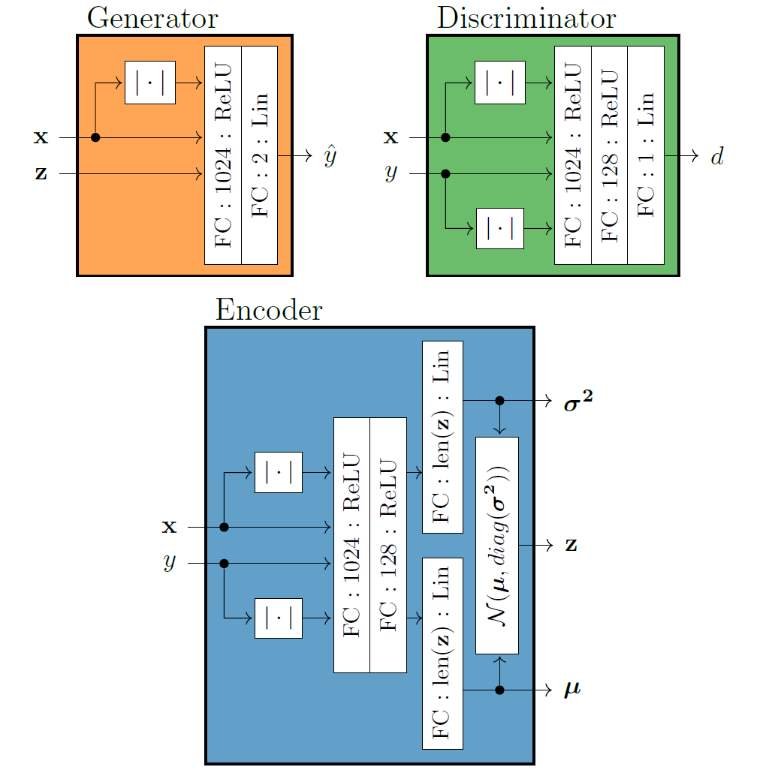Figure 5: GAN Architecture. Source: Adapted from 

## IV. Results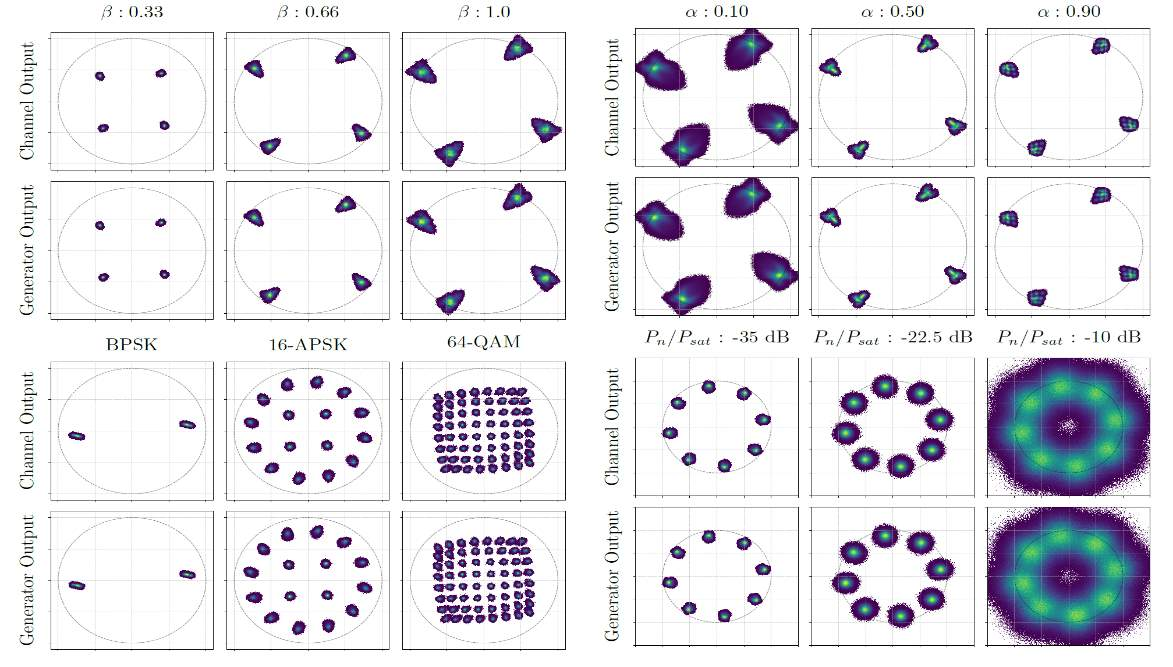Figure 6: Generator Results for default channel (a). Source: Adapted from 

The Generator network is evaluated by comparing the marginal probability density function of the channel with the one created by a pre-trained generator network. The approximate probability density function is represented by a 2D histogram and is shown in Figure 6. They sample the transmit signals from a mixed bag of constellations randomly, the normalized power of which can be calculated as shown below, 

where PAPR represents the peak to average power ratio and  is an amplitude scale factor which is one of the controlling variables as shown in Figure 6.

All the input symbol stream are interpolated using a 129 tap RRC pulse-shaping filter and use a roll-off factor α. In the top left plot of Figure 6., they try to examine the results of keeping all the other things constant and varying $\beta$ while simultaneously sending QPSK signal to both the channel and the generator. The power amplification increases along with $\beta$ and is driven into saturation causing inter symbol interference and distorting the output, which shows that the generator is able to generalize and learn channel response over a range of power values.

In the bottom left part of Figure 6, they keep the $\beta$ value constant while varying the input constellation and the generator is able to perform decently to match the symbols. In the top right plot of figure 6. they keep all the other variables constant and vary the roll-off factor α and train different generators, which all learn the various channel responses correctly.

Finally, the last plot shows the generator performance for different signal-to-noise ratios and its evident that the generator perform better in lower ratios.

Figure 7. shows the performance of the generator for the other 3 channels (b, c and d), Notice that the filter created an Additive white gaussian noise like distribution around the symbols. but the generator is still able to generalize for cases with multi-path elements and phase noise.

(b)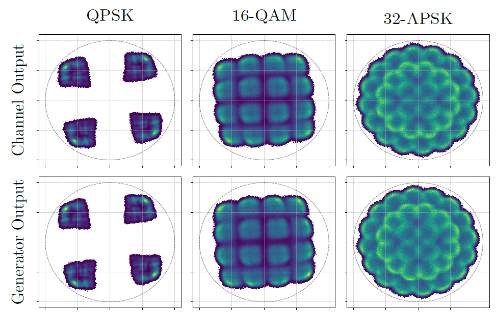(c)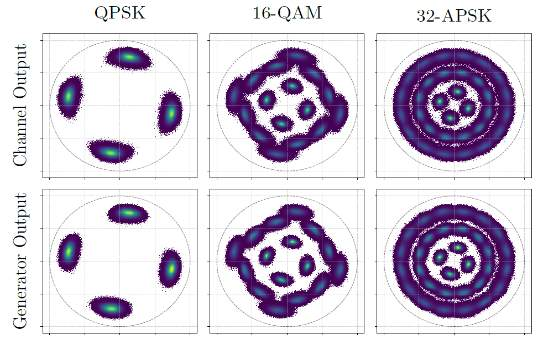(d)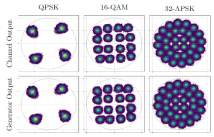Figure 7: Generator performance for Channels (b), (c) and (d). Source: Adapted from 

## V. Conclusion

Generative Adversarial Networks are a fantastic tool to simulate complex physical channel environments and re-emphasize their importance in varied real-world applications where no equivalent models exist. The generative nature of these neural networks in estimating channels without too many assumptions and constraints on the medium is what makes them powerful. We saw an example which showcased a GAN that could estimate arbitrary symbols in a combination of environments with memory effects, non-linearities and non-gaussian elements.

## VI. References

 Kwan-Yuet (Stephen) Ho. “Generative-Discriminative Pairs”. Internet: https://datawarrior.wordpress.com/2016/05/08/generative-discriminative-pairs/ , May 6, 2016 [Mar. 3, 2020].

. Goodfellow, Ian & Pouget-Abadie, Jean & Mirza, Mehdi & Xu, Bing & Warde-Farley, David & Ozair, Sherjil & Courville, Aaron & Bengio, Y.. (2014). Generative Adversarial Nets. ArXiv.

 T. J. O’Shea, T. Roy and N. West, "Approximating the Void: Learning Stochastic Channel Models from Observation with Variational Generative Adversarial Networks," 2019 International Conference on Computing, Networking and Communications (ICNC), Honolulu, HI, USA, 2019, pp. 681-686.

 A. Smith and J. Downey, "A Communication Channel Density Estimating Generative Adversarial Network," 2019 IEEE Cognitive Communications for Aerospace Applications Workshop (CCAAW), Cleveland, OH, USA, 2019, pp. 1-7.

 S. Lazebnik. “Generative adversarial networks”. Lecture link: http://slazebni.cs.illinois.edu/fall18/lec13_gan.pdf . Nov. 6, 2018 [Mar. 3, 2020].

## Related Services

View all

Related Content

All Tags

Content relating to: "Physiology"

Physiology is related to biology, and is the study of living organisms and how they function. Physiology covers all living organisms, exploring how the body performs basic functions in relation to physics and chemistry.

Related Articles

### DMCA / Removal Request

If you are the original writer of this dissertation and no longer wish to have your work published on the UKDiss.com website then please:

Related Services